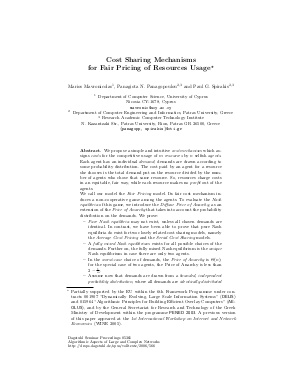Document# Cost Sharing Mechanisms for Fair Pricing of Resources Usage

### Authors Marios Mavronicolas, Panagiota Panagopoulou, Paul G. Spirakis## File

DagSemProc.05361.5.pdf
• Filesize: 289 kB
• 18 pages

## Cite As

Marios Mavronicolas, Panagiota Panagopoulou, and Paul G. Spirakis. Cost Sharing Mechanisms for Fair Pricing of Resources Usage. In Algorithmic Aspects of Large and Complex Networks. Dagstuhl Seminar Proceedings, Volume 5361, pp. 1-18, Schloss Dagstuhl - Leibniz-Zentrum für Informatik (2006)
https://doi.org/10.4230/DagSemProc.05361.5

## Abstract

We propose a simple and intuitive cost mechanism which assigns costs for the competitive usage of \$m\$ resources by \$n\$ selfish agents. Each agent has an individual demand; demands are drawn according to some probability distribution. The cost paid by an agent for a resource she chooses is the total demand put on the resource divided by the number of agents who chose that same resource. So, resources charge costs in an equitable, fair way, while each resource makes no profit out of the agents. We call our model the Fair Pricing model. Its fair cost mechanism induces a non-cooperative game among the agents. To evaluate the Nash equilibria of this game, we introduce the Diffuse Price of Anarchy, as an extension of the Price of Anarchy that takes into account the probability distribution on the demands. We prove: (1) Pure Nash equilibria may not exist, unless all chosen demands are identical. In contrast, we have been able to prove that pure Nash equilibria do exist for two closely related cost sharing models, namely the Average Cost Pricing and the Serial Cost Sharing models. (2) A fully mixed Nash equilibrium exists for all possible choices of the demands. Further on, the fully mixed Nash equilibrium is the unique Nash equilibrium in case there are only two agents. (3) In the worst-case choice of demands, the Price of Anarchy is \$Theta (n)\$; for the special case of two agents, the Price of Anarchy is less than \$2 - frac{1}{m}\$. (4) Assume now that demands are drawn from a bounded, independent probability distribution, where all demands are identically distributed and each is at most a (universal for the class) constant times its expectation. Then, the Diffuse Price of Anarchy is at most that same constant, which is just 2 when each demand is distributed symmetrically around its expectation.
##### Keywords
• Cost Sharing
• Diffuse Price of Anarchy
• Fair Pricing
• Resources

## Metrics

• Access Statistics
• Total Accesses (updated on a weekly basis)
0